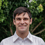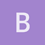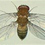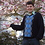This is an archived static version of the original phylobabble.org discussion site.

# Matrix strategies to avoid rate matrix exponentiationematsen

Continuing the discussion from [Paper] Phylogenetic Stochastic Mapping without Matrix Exponentiation:jgsumner

Correct me if I’m wrong but I don’t think it’s right to say that you are not computing a matrix exponential because you are doing it via diagonalisation. This is just one way (of many) of calculating a matrix exp. There is a semi-famous paper on this: http://www.cs.cornell.edu/cv/researchpdf/19ways+.pdf

It would be a different story if P was the same for all rate matrices (as it is for the K3ST model where P=H , the Hadamard matrix), but in general P changes as the rate matrix changes. I’m interested in generalisations of this situation to models more complicated then K3ST. That’s essentially what I mean by “explicit formulas”.

I see what uniformization is now. Actually a bit of a coincidence because we were considering exactly of this over our summer break (southern hemisphere) but of course have done nothing about it. It’s a very interesting way of thinking about a CTMC.nicolas_lartill

Maybe we can quibble on the exact wording, but in any case, I want to emphasize that there are important algorithmic differences here, depending on how you do it:

if you do:

(1) L_up = [P [ exp( t D) P^{-1}] L_down

then it is in O(n^3), whereas if you do

(2) L_up = P [ exp( t D) [ P^{-1} L_down] ]

it is O(n^2)

More importantly, if you have a large tree and the same rate matrix for all branches of the tree, and if you use (1), then you have to compute exp(tQ) for all branches. Whereas you have to compute the diagonalization only once for all branches.

So, there is indeed an important difference in terms of computational complexity.jgsumner

As you point out, the important thing is that, for each Q, P only needs to be computed once for the whole tree, so the computation of P itself is not included in the (per branch) complexity. This only works if Q is fixed over the whole tree though. What if Q (and hence D) is changing over the tree though? Then the complexity of computing P has to be included for every branch. That’s why I point to the K3ST example. In that case P=H, no matter what Q is.

I think my interest in this is fairly idiosyncratic. I don’t want to distract from the main point of discussion.ematsen

@jgsumner and @nicolas_lartill I moved this discussion here. I know @Alexis_RAxML has some nice strategies along these lines.nicolas_lartill

yes exactly: it all depends on the exact situation. It is just a question of the relative cost of matrix diagonalization, exponentiation, and likelihood computation. The relative weights of those things really depend on the model.

In this respect, the work of @vminin is particularly interesting when you have different rate matrices for each site and/or each branchnicolas_lartill

yes, you are right. This is kind of a side track, in the other topic

thanksvminin

The fact that @nicolas_lartill finds our work interesting makes the outcome of the formal peer-review totally irrelevant for me, seriously!jgsumner

By the way, thanks for pointing this out (option (1) vs. (2) that is). It’s a very nice subtle thing I’d never fully appreciated before.